# 前端js深入理解贝塞尔曲线原理附代码

n阶贝塞尔曲线（n+1个控制点）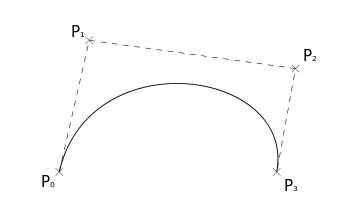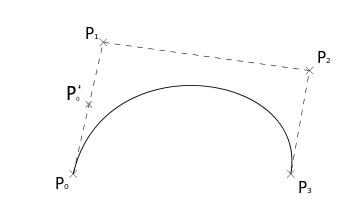2.接下来对每一条线段做同样的操作，得到三个控制点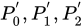，如下图所示。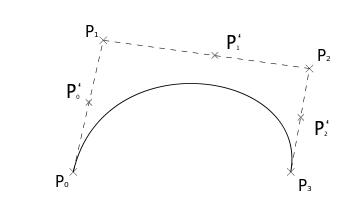3.然后对这三个控制点重复第1步操作，得出两个控制点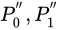，如下图所示。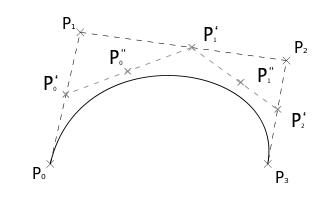4. 最后再使用同样的方法可以得到，最终的一个点，如下图所示，此时这个点就是贝塞尔曲线上的一个点。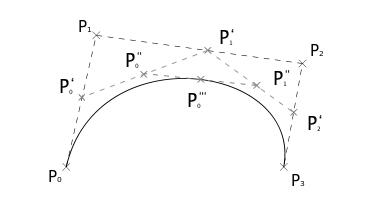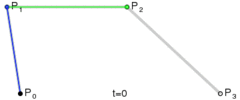1、一阶贝塞尔曲线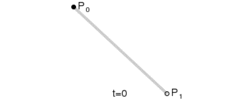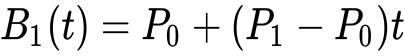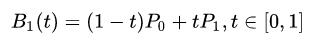2、二阶贝塞尔曲线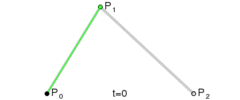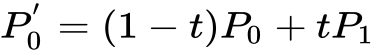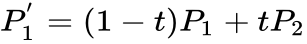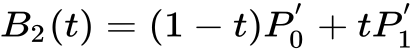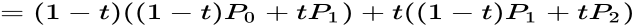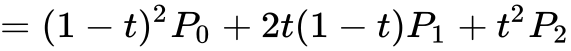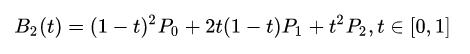3、三阶贝塞尔曲线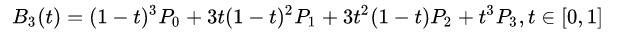4、多阶贝塞尔曲线5、如何实现一个类似CSS中easing属性的三阶贝塞尔曲线构造函数？

CSS中的easing贝塞尔曲线有一个特点，那就是起点和终点是固定的，也就是分别是[0,0],[1,1]。所以未知的点就只有两个，也就是需要传入四个值，并且这四个值的范围需要在[0,1]内。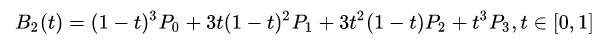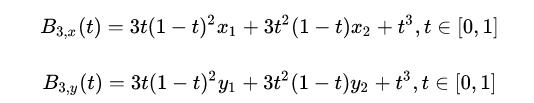class CubicBezier {
constructor(x1, y1, x2, y2) {
this.controlPoints = [x1, y1, x2, y2];
}
}

class CubicBezier {
constructor(x1, y1, x2, y2) {
this.controlPoints = [x1, y1, x2, y2];
}

getCoord(t) {
// 如果t取值不在0到1之间，则终止操作
if (t > 1 || t < 0) return;
const _t = 1 - t;
const [ x1, y1, x2, y2 ] = this.controlPoints;
const coefficient1 = 3 * t * Math.pow(_t, 2);
const coefficient2 = 3 * _t * Math.pow(t, 2);
const coefficient3 = Math.pow(t, 3);
const px = coefficient1 * x1 + coefficient2 * x2 + coefficient3;
const py = coefficient1 * y1 + coefficient2 * y2 + coefficient3;
// 结果只保留三位有效数字
return [parseFloat(px.toFixed(3)), parseFloat(py.toFixed(3))];
}
}

1.先获取离需要求值点最近的两个点。
2.然后通过这两个点可以得到一个直线方程。
3.最后通过将x轴坐标传入直线方程中，就可以近似求得y轴坐标值了。

class CubicBezier {
constructor(x1, y1, x2, y2) {
const precision = 100;
this.controlPoints = [x1, y1, x2, y2];
this.coords = this.getCoordsArray(precision);
}

getCoord(t) {
// 如果t取值不在0到1之间，则终止操作
if (t > 1 || t < 0) return;
const _t = 1 - t;
const [ x1, y1, x2, y2 ] = this.controlPoints;
const coefficient1 = 3 * t * Math.pow(_t, 2);
const coefficient2 = 3 * _t * Math.pow(t, 2);
const coefficient3 = Math.pow(t, 3);
const px = coefficient1 * x1 + coefficient2 * x2 + coefficient3;
const py = coefficient1 * y1 + coefficient2 * y2 + coefficient3;
// 结果只保留三位有效数字
return [parseFloat(px.toFixed(3)), parseFloat(py.toFixed(3))];
}

getCoordsArray(precision) {
const step = 1 / (precision + 1);
const result = [];
for (let t = 0; t <= precision + 1; t++) {
result.push(this.getCoord(t * step));
}
this.coords = result;
return result;
}

getY(x) {
if (x >= 1) return 1;
if (x <= 0) return 0;
let startX = 0;
for (let i = 0; i < this.coords.length; i++) {
if (this.coords[i] >= x) {
startX = i;
break;
}
}
const axis1 = this.coords[startX];
const axis2 = this.coords[startX - 1];
const k = (axis2 - axis1) / (axis2 - axis1);
const b = axis1 - k * axis1;
// 结果也只保留三位有效数字
return parseFloat((k * x + b).toFixed(3));
}
}

const cubicBezier = new CubicBezier(0.3, 0.1, 0.3, 1);
cubicBezier.getY(0.1); // 0.072
cubicBezier.getY(0.7); // 0.931复制代码

React是前端最火的框架之一，就业薪资很高，本课程教您如何快速学会React并应用到实战，对正在工作当中或打算学习React高薪就业的你来说，那么这门课程便是你手中的葵花宝典。
React是目前最火的前端框架，就业薪资很高，本课程教您如何快速学会React并应用到实战，教你如何解决内存泄漏，常用UI库的使用，自己封装组件，正式上线白屏问题，性能优化等。对正在工作当中或打算学习React高薪就...
HTML5基础入门视频教程，教学思路清晰，简单易学必会。适合人群：创业者，只要会打字，对互联网编程感兴趣都可以学。课程概述：该课程主要讲解HTML(学习HTML5的必备基础语言)、CSS3、Javascript(学习...
jquery视频教程从入门到精通，课程主要包含：jquery选择器、jquery事件、jquery文档操作、动画、Ajax、jquery插件的制作、jquery下拉无限加载插件的制作等等......
React和VUE是目前最火的前端框架，就业薪资很高，本课程教您如何快速学会React和VUE并应用到实战，教你如何解决内存泄漏，常用库的使用，自己封装组件，正式上线白屏问题，性能优化等。对正在工作当中或打算学习Re...
VUE视频教程是目前最火的前端框架之一，就业薪资很高，本课程教您如何快速学会VUE并应用到实战，教你如何解决内存泄漏，常用UI库的使用，自己封装组件，正式上线白屏问题，性能优化等。对正在工作当中或打算学习VUE高薪就...